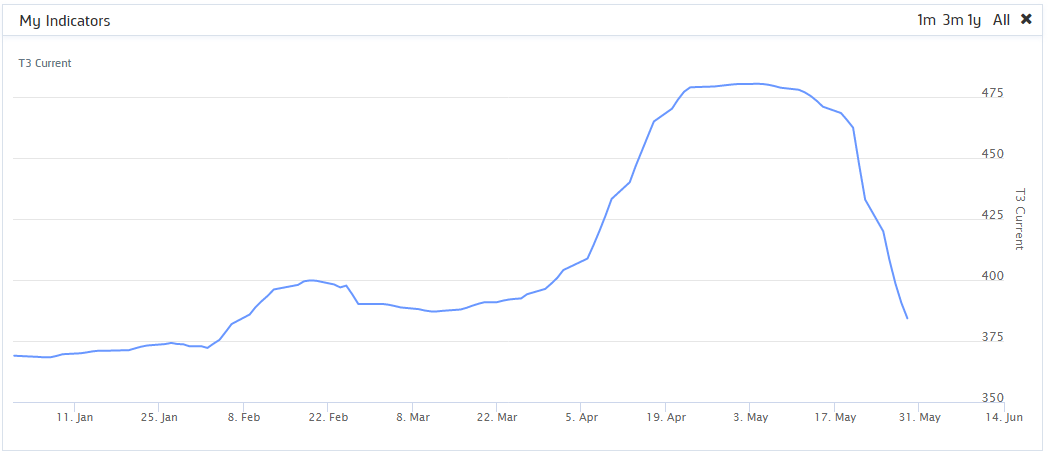# Indicator Reference

## T3 Moving Average

### Introduction

This indicator computes the T3 Moving Average (T3). The T3 Moving Average is calculated with the following formula: EMA1(x, Period) = EMA(x, Period) EMA2(x, Period) = EMA(EMA1(x, Period),Period) GD(x, Period, volumeFactor) = (EMA1(x, Period)*(1+volumeFactor)) - (EMA2(x, Period)* volumeFactor) T3 = GD(GD(GD(t, Period, volumeFactor), Period, volumeFactor), Period, volumeFactor);

### Create Manual Indicators

You can manually create a T3MovingAverage indicator, so it doesn’t automatically update. Manual indicators let you update their values with any data you choose. The following reference table describes the T3MovingAverage constructor.

### T3MovingAverage()1/2

            T3MovingAverage QuantConnect.Indicators.T3MovingAverage (
string    name,
int       period,
*decimal  volumeFactor
)


Initializes a new instance of the T3MovingAverage class using the specified name and period.

### T3MovingAverage()2/2

            T3MovingAverage QuantConnect.Indicators.T3MovingAverage (
int       period,
*decimal  volumeFactor
)


Initializes a new instance of the T3MovingAverage class using the specified period.

### Update Manual Indicators

You can update the indicator automatically or manually.

#### Automatic Update

To register a manual indicator for automatic updates with the security data, call the RegisterIndicator method.


private T3MovingAverage _t3;

// In Initialize()
_t3 = new T3MovingAverage(name, period);
_t3.Updated += IndicatorUpdateMethod;

RegisterIndicator(symbol, _t3, Resolution.Daily);

// In IndicatorUpdateMethod()
{
var indicatorValue = _t3.Current.Value;
}

# In Initialize()
self.t3 = T3MovingAverage(name, period)
self.t3.Updated += self.IndicatorUpdateMethod

self.RegisterIndicator(symbol, self.t3, Resolution.Daily)

# In IndicatorUpdateMethod()
indicator_value = self.t3.Current.Value

To customize the data that automatically updates the indicator, see Custom Indicator Periods and Custom Indicator Values.

#### Manual Update

Updating your indicator manually enables you to control when the indicator is updated and what data you use to update it. To manually update the indicator, call the Update method with time/decimal pair. The indicator will only be ready after you prime it with enough data.


private T3MovingAverage _t3;
private Symbol symbol;

// In Initialize()
_t3 = new T3MovingAverage(period);

// In OnData()
if (data.ContainsKey(_symbol))
{
_t3.Update(data[symbol].EndTime, data[symbol].High);
}
{
var indicatorValue = _t3.Current.Value;
}

# In Initialize()
self.t3 = T3MovingAverage(period)

# In OnData()
if data.ContainsKey(self.symbol):
self.t3.Update(data[self.symbol].EndTime, data[self.symbol].High)
indicator_value = self.t3.Current.Value

### Create Automatic Indicators

The T3 method creates an T3MovingAverage indicator, sets up a consolidator to update the indicator, and then returns the indicator so you can use it in your algorithm.

The following reference table describes the T3 method:

### T3()1/1

            T3MovingAverage QuantConnect.Algorithm.QCAlgorithm.T3 (
Symbol                           symbol,
Int32                            period,
*Decimal                         volumeFactor,
*Nullable<Resolution>      resolution,
*Func<IBaseData, Decimal>  selector
)


Creates a new T3MovingAverage indicator.

If you don't provide a resolution, it defauls to the security resolution. If you provide a resolution, it must be greater than or equal to the resolution of the security. For instance, if you subscribe to hourly data for a security, you should update its indicator with data that spans 1 hour or longer.

### Get Indicator Values

To get the value of the indicator, use its Current.Value attribute.

private T3MovingAverage _t3;

// In Initialize()
_t3 = T3(symbol, period);

// In OnData()
{
var current = _t3.Current.Value;
}
# In Initialize()
self.t3 = self.T3(symbol, period)

# In OnData()
current = self.t3.Current.Value


### Visualization

To plot indicator values, in the OnData event handler, call the Plot method.

private T3MovingAverage _t3;

// In Initialize()
_t3 = T3(symbol, period);

// In OnData()
{
Plot("My Indicators", "t3movingaverage", _t3.Current);
}
# In Initialize()